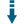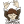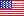# Lexographical PHP Permutations Generator: Generate permutations of characters of a stringDownload .zip
 InfoDocumentationView files (8)Download .zip Reputation Support forum Blog Links
 Ratings Unique User Downloads Download Rankings Not enough user ratings Total: 92 All time: 9,775 This week: 165Version License PHP version Categories permutations 1.0 GNU General Publi... 5 Algorithms, PHP 5, Text processing
Description Author

This class can generate permutations of characters of a string.

It takes a given string and returns a new string with the same characters in a exchanged order.

The class can return all the permutation of a text string and iterate to next, previous, first and last permutations in the whole permutations sequence.Performance Level
Name: Classes: Jelle Sebreghts `` 7 packages by Jelle SebreghtsUnited States 33 1486 220 in United States14612 in United StatesInnovation awardNominee: 4x

Details

Generates (lexographical) permutations.

``````use Jelle_S\Util\Permutation\LexographicalPermutation;
\$number = 12345;
\$next = LexographicalPermutation::getNextPermutation(\$number);
print "The next lexographical permutation of {\$number} is {\$next}.\n";

\$number = 32154;
\$previous = LexographicalPermutation::getPreviousPermutation(\$number);
print "The previous lexographical permutation of {\$number} is {\$previous}.\n";

\$first = LexographicalPermutation::getFirstPermutation(\$number);
print "The first lexographical permutation of {\$number} is {\$first}.\n";

\$last = LexographicalPermutation::getLastPermutation(\$number);
print "The last lexographical permutation of {\$number} is {\$last}.\n";

\$number = 3124;
\$all = print_r(LexographicalPermutation::getAllPermutations(\$number), TRUE);
print "All lexographical permutations of {\$number} are \n{\$all}\n";
``````

Output:

``````The next lexographical permutation of 12345 is 12354.
The previous lexographical permutation of 32154 is 32145.
The first lexographical permutation of 32154 is 12345.
The last lexographical permutation of 32154 is 54321.
All lexographical permutations of 3124 are
Array
(
 => 1234
 => 1243
 => 1324
 => 1342
 => 1423
 => 1432
 => 2134
 => 2143
 => 2314
 => 2341
 => 2413
 => 2431
 => 3124
 => 3142
 => 3214
 => 3241
 => 3412
 => 3421
 => 4123
 => 4132
 => 4213
 => 4231
 => 4312
 => 4321
)
``````Files
File Role Descriptionsrc (1 file)tests (1 file).codeclimate.yml Data Auxiliary data.travis.yml Data Auxiliary datacomposer.json Data Auxiliary dataLICENSE Lic. License textphpunit.xml Data Auxiliary dataREADME.md Doc. DocumentationFiles / src
File Role DescriptionLexographicalPermutation.php Class Class sourceFiles / tests
File Role DescriptionLexographicalPermutationTest.php Class Class source

 All time: 9,775 This week: 165About usAdvertise on this siteSite mapNewsletterStatisticsSite tipsPrivacy policyContact
For more information send a message to `info at phpclasses dot org`.